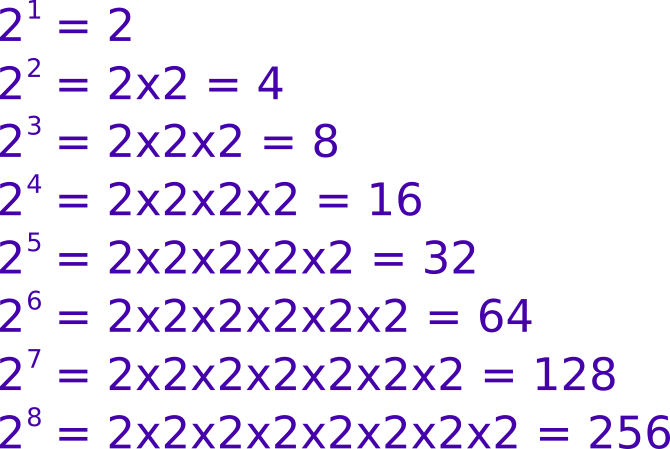# Powers of two

Martin McBride, 2016-11-23
Tags powers of two bit byte
Categories data representation binary numbers

Computers use binary (base 2), so it isn't surprising that powers of two occur quite often (in much the same way that hundreds, thousands and millions crop up in denary all the time).

You will get very used to seeing these numbers, and it is useful to be familiar with them.

## Powers of 2 up to 8

Just to recap 2 to the power n means 2 multiplied by itself n times. For example 22 (2 to the power 2, often called 2 squared) is 2x2, which equals 4. 24 (2 to the power 4) is 2x2x2x2, which equals 16. Here is a table of all the values up to 8:## Powers of 2 up to 16

Now that you know how the powers are calculated, here is a more concise table which just gives the answer, for powers up to 16:## Uses

A byte has 8 bits. Each bit has two possible values, 0 or 1. This means that a byte has 256 possible value: 2x2x2x2x2x2x2x2 or 2 to the power 8.

If we use a byte to store a number, the range of values is 0 to 255 (ie one less than 2 to the power 8).

We can use this idea to calculate the range of other word sizes. For example, a nibble has 4 bits. So there are 16 possible combinations, and it can hold a number between 0 to 15.

A word has 16 bits. So (from the table) there are 65536 possible combinations, and it can hold a number between 0 to 65535.